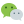# 递归实例

## 德罗斯特效应# 递归实现

``````#include <stdio.h>
int factorial(int n) /*外部定义阶乘函数*/
{
int m;
if (n < 0)  /*注意要给函数设置一个递归结束条件*/
{
printf("输入错误！");
return 0;
}
else if (n == 1)
{
m = 1;
}
else
{
m = n * factorial(n-1); /*调用函数factorial()自身*/
}
return m;
}
int main()
{
printf("%d", factorial(5));

}
``````

### 评论：微信公众号：程序骑士